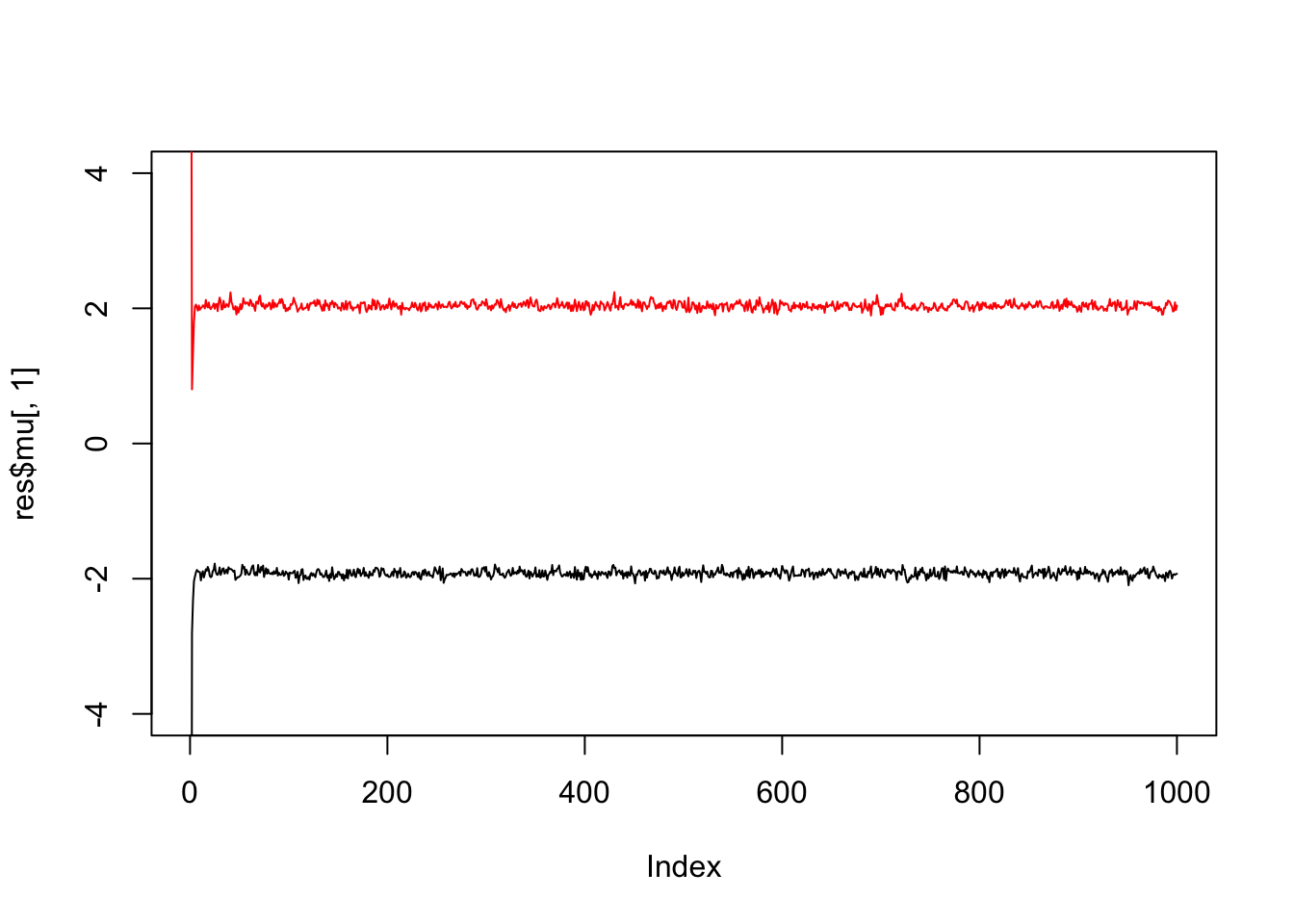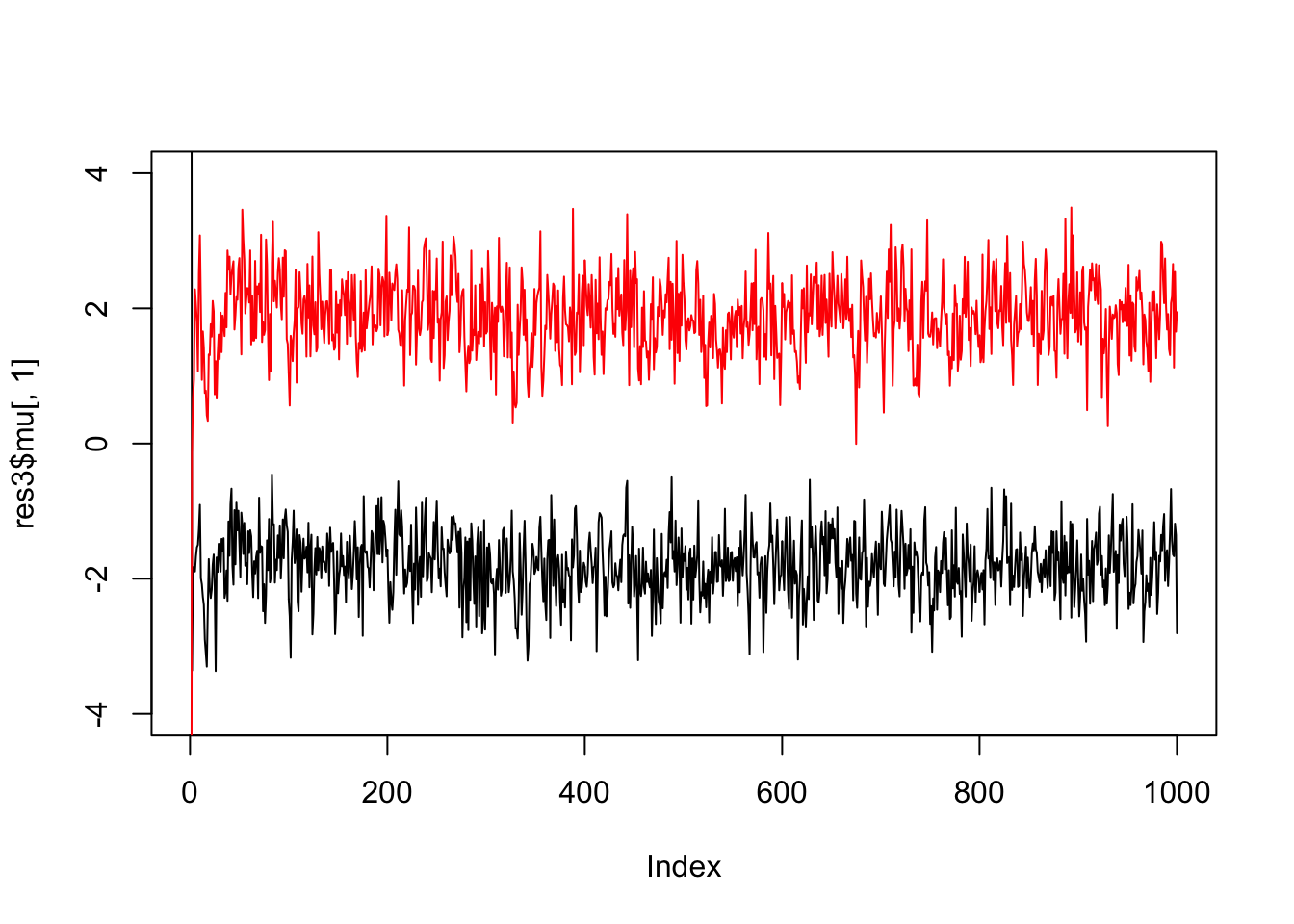Last updated: 2021-02-04

Checks: 7 0

Knit directory: fiveMinuteStats/analysis/

This reproducible R Markdown analysis was created with workflowr (version 1.6.2). The Checks tab describes the reproducibility checks that were applied when the results were created. The Past versions tab lists the development history.

Great! Since the R Markdown file has been committed to the Git repository, you know the exact version of the code that produced these results.

Great job! The global environment was empty. Objects defined in the global environment can affect the analysis in your R Markdown file in unknown ways. For reproduciblity it’s best to always run the code in an empty environment.

The command set.seed(12345) was run prior to running the code in the R Markdown file. Setting a seed ensures that any results that rely on randomness, e.g. subsampling or permutations, are reproducible.

Great job! Recording the operating system, R version, and package versions is critical for reproducibility.

Nice! There were no cached chunks for this analysis, so you can be confident that you successfully produced the results during this run.

Great job! Using relative paths to the files within your workflowr project makes it easier to run your code on other machines.

Great! You are using Git for version control. Tracking code development and connecting the code version to the results is critical for reproducibility.

The results in this page were generated with repository version e8976cf. See the Past versions tab to see a history of the changes made to the R Markdown and HTML files.

Note that you need to be careful to ensure that all relevant files for the analysis have been committed to Git prior to generating the results (you can use wflow_publish or wflow_git_commit). workflowr only checks the R Markdown file, but you know if there are other scripts or data files that it depends on. Below is the status of the Git repository when the results were generated:


Ignored files:
Ignored:    .Rhistory
Ignored:    .Rproj.user/
Ignored:    analysis/.Rhistory
Ignored:    analysis/bernoulli_poisson_process_cache/

Untracked files:
Untracked:  _workflowr.yml
Untracked:  analysis/CI.Rmd
Untracked:  analysis/gibbs_structure.Rmd
Untracked:  analysis/libs/
Untracked:  analysis/results.Rmd
Untracked:  analysis/shiny/tester/

Unstaged changes:
Modified:   analysis/LR_and_BF.Rmd
Modified:   analysis/MH-examples1.Rmd
Modified:   analysis/MH_intro.Rmd
Deleted:    analysis/r_simplemix_extended.Rmd



Note that any generated files, e.g. HTML, png, CSS, etc., are not included in this status report because it is ok for generated content to have uncommitted changes.

These are the previous versions of the repository in which changes were made to the R Markdown (analysis/gibbs2.Rmd) and HTML (docs/gibbs2.html) files. If you’ve configured a remote Git repository (see ?wflow_git_remote), click on the hyperlinks in the table below to view the files as they were in that past version.

File Version Author Date Message
Rmd e8976cf Matthew Stephens 2021-02-04 workflowr::wflow_publish(“analysis/gibbs2.Rmd”)
html 5f62ee6 Matthew Stephens 2019-03-31 Build site.
Rmd 0cd28bd Matthew Stephens 2019-03-31 workflowr::wflow_publish(all = TRUE)
html 34bcc51 John Blischak 2017-03-06 Build site.
Rmd 5fbc8b5 John Blischak 2017-03-06 Update workflowr project with wflow_update (version 0.4.0).
Rmd 391ba3c John Blischak 2017-03-06 Remove front and end matter of non-standard templates.
html 8e61683 Marcus Davy 2017-03-03 rendered html using wflow_build(all=TRUE)
html c3b365a John Blischak 2017-01-02 Build site.
Rmd 67a8575 John Blischak 2017-01-02 Use external chunk to set knitr chunk options.
Rmd 5ec12c7 John Blischak 2017-01-02 Use session-info chunk.
Rmd a8c9d57 stephens999 2016-09-04 correct misleading names in gibbs2 example
Rmd 6bb4560 stephens999 2016-05-12 add gibbs sampling example

# Pre-requisites

You should know about Gibbs sampling and mixture models, and be familiar with Bayesian inference for the normal mean and for the two class problem.

# Overview

We consider using Gibbs sampling to perform inference for a normal mixture model, $X_1,\dots,X_n \sim f(\cdot)$ where $f(\cdot) = \sum_{k=1}^K \pi_k N(\cdot; \mu_k,1).$ Here $$\pi_1,\dots,\pi_K$$ are non-negative and sum to 1, and $$N(\cdot;\mu,\sigma^2)$$ denotes the density of the $$N(\mu,\sigma^2)$$ distribution.

Recall the latent variable representation of this model: $\Pr(Z_j = k) = \pi_k$ $X_j | Z_j = k \sim N(\mu_k,1)$

To illustrate, let’s simulate data from this model:

set.seed(33)

# generate from mixture of normals
#' @param n number of samples
#' @param pi mixture proportions
#' @param mu mixture means
#' @param s mixture standard deviations
rmix = function(n,pi,mu,s){
z = sample(1:length(pi),prob=pi,size=n,replace=TRUE)
x = rnorm(n,mu[z],s[z])
return(x)
}
x = rmix(n=1000,pi=c(0.5,0.5),mu=c(-2,2),s=c(1,1))
hist(x)Version Author Date
5f62ee6 Matthew Stephens 2019-03-31
c3b365a John Blischak 2017-01-02

# Gibbs sampler

Suppose we want to inference for the parameters $$\mu,\pi$$. That is, we want to sample from $$p(\mu,\pi | x)$$. We can use a Gibbs sampler. However, to do this we have to augment the space to sample from $$p(z,\mu,\pi | x)$$, not only $$p(\mu,\pi | x)$$.

Here is the algorithm in outline:

• sample $$\mu$$ from $$\mu | x, z, \pi$$
• sample $$\pi$$ from $$\pi | x, z, \mu$$
• sample $$z$$ from $$z | x, \pi, \mu$$

The point here is that all of these conditionals are easy to sample from.

# Code

  normalize = function(x){return(x/sum(x))}

#' @param x an n vector of data
#' @param pi a k vector
#' @param mu a k vector
sample_z = function(x,pi,mu){
dmat = outer(mu,x,"-") # k by n matrix, d_kj =(mu_k - x_j)
p.z.given.x = as.vector(pi) * dnorm(dmat,0,1)
p.z.given.x = apply(p.z.given.x,2,normalize) # normalize columns
z = rep(0, length(x))
for(i in 1:length(z)){
z[i] = sample(1:length(pi), size=1,prob=p.z.given.x[,i],replace=TRUE)
}
return(z)
}

#' @param z an n vector of cluster allocations (1...k)
#' @param k the number of clusters
sample_pi = function(z,k){
counts = colSums(outer(z,1:k,FUN="=="))
pi = gtools::rdirichlet(1,counts+1)
return(pi)
}

#' @param x an n vector of data
#' @param z an n vector of cluster allocations
#' @param k the number o clusters
#' @param prior.mean the prior mean for mu
#' @param prior.prec the prior precision for mu
sample_mu = function(x, z, k, prior){
df = data.frame(x=x,z=z)
mu = rep(0,k)
for(i in 1:k){
sample.size = sum(z==i)
sample.mean = ifelse(sample.size==0,0,mean(x[z==i]))

post.prec = sample.size+prior$prec post.mean = (prior$mean * prior$prec + sample.mean * sample.size)/post.prec mu[i] = rnorm(1,post.mean,sqrt(1/post.prec)) } return(mu) } gibbs = function(x,k,niter =1000,muprior = list(mean=0,prec=0.1)){ pi = rep(1/k,k) # initialize mu = rnorm(k,0,10) z = sample_z(x,pi,mu) res = list(mu=matrix(nrow=niter, ncol=k), pi = matrix(nrow=niter,ncol=k), z = matrix(nrow=niter, ncol=length(x))) res$mu[1,]=mu
res$pi[1,]=pi res$z[1,]=z
for(i in 2:niter){
pi = sample_pi(z,k)
mu = sample_mu(x,z,k,muprior)
z = sample_z(x,pi,mu)
res$mu[i,] = mu res$pi[i,] = pi
res$z[i,] = z } return(res) } Try the Gibbs sampler on the data simulated above. We see it quickly moves to a part of the space where the mean parameters are near their true values (-2,2).  res = gibbs(x,2) plot(res$mu[,1],ylim=c(-4,4),type="l")
lines(res$mu[,2],col=2)Version Author Date 5f62ee6 Matthew Stephens 2019-03-31 c3b365a John Blischak 2017-01-02 If we simulate data with fewer observations we should see more uncertainty  x = rmix(100,c(0.5,0.5),c(-2,2),c(1,1)) res2 = gibbs(x,2) plot(res2$mu[,1],ylim=c(-4,4),type="l")
lines(res2$mu[,2],col=2)Version Author Date 5f62ee6 Matthew Stephens 2019-03-31 c3b365a John Blischak 2017-01-02 And fewer observations still…  x = rmix(10,c(0.5,0.5),c(-2,2),c(1,1)) res3 = gibbs(x,2) plot(res3$mu[,1],ylim=c(-4,4),type="l")
lines(res3$mu[,2],col=2)Version Author Date 5f62ee6 Matthew Stephens 2019-03-31 c3b365a John Blischak 2017-01-02 And we can get credible intervals (CI) from these samples (discard the first few samples as “burn-in”). For example, to get 90% posterior CIs for the mean parameters:  quantile(res3$mu[-(1:10),1],c(0.05,0.95))
       5%       95%
-2.644896 -1.004009 
  quantile(res3\$mu[-(1:10),2],c(0.05,0.95))
       5%       95%
0.9400428 2.7773584 

sessionInfo()
R version 3.6.0 (2019-04-26)
Platform: x86_64-apple-darwin15.6.0 (64-bit)
Running under: macOS  10.16

Matrix products: default
BLAS:   /Library/Frameworks/R.framework/Versions/3.6/Resources/lib/libRblas.0.dylib
LAPACK: /Library/Frameworks/R.framework/Versions/3.6/Resources/lib/libRlapack.dylib

locale:
 en_US.UTF-8/en_US.UTF-8/en_US.UTF-8/C/en_US.UTF-8/en_US.UTF-8

attached base packages:
 stats     graphics  grDevices utils     datasets  methods   base

loaded via a namespace (and not attached):
 Rcpp_1.0.6       rstudioapi_0.11  whisker_0.4      knitr_1.29
 magrittr_1.5     workflowr_1.6.2  R6_2.4.1         rlang_0.4.8
 stringr_1.4.0    tools_3.6.0      xfun_0.16        git2r_0.27.1
 gtools_3.8.2     htmltools_0.5.0  ellipsis_0.3.1   yaml_2.2.1
 digest_0.6.27    rprojroot_1.3-2  tibble_3.0.4     lifecycle_0.2.0
 crayon_1.3.4     later_1.1.0.1    vctrs_0.3.4      fs_1.5.0
 promises_1.1.1   glue_1.4.2       evaluate_0.14    rmarkdown_2.3
 stringi_1.4.6    compiler_3.6.0   pillar_1.4.6     backports_1.1.10
 httpuv_1.5.4     pkgconfig_2.0.3 

This site was created with R Markdown